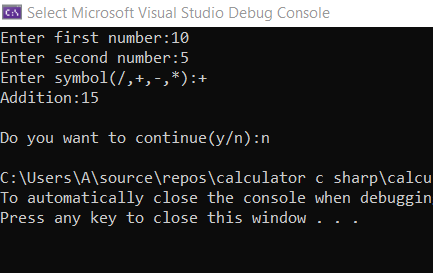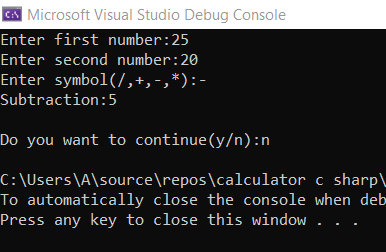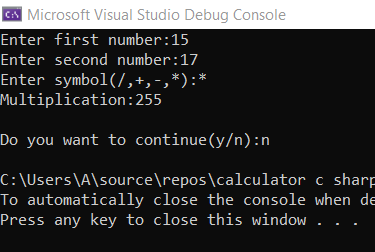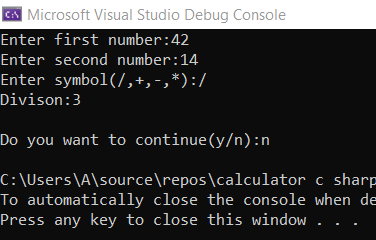# How to Make a Calculator in C# ?

• Last Updated : 20 Jul, 2022

C# is an object-oriented, modern programming language that was created by Microsoft. It runs on the .NET Framework. C# is very close to C/C++ and Java programming languages. In this article, we will learn how to create a calculator in  C#.

### Basic Functions of Calculator:

• Difference between two numbers.
• Product of two numbers.
• Division of two numbers.

### Approach:

• Declare local variables num1 and num2 for two numeric values.
• Enter the choice.
• Takes two numbers, num1, and num2.
• Display the operation result.
• Exit

Example:

## C#

 `// Implementing a calculator in``// C# using switch statement.``using` `System;``using` `System.Text;``using` `System.Threading.Tasks;` `namespace` `calculator_c_sharp``{``    ``class` `Program``    ``{``        ``static` `void` `Main(``string``[] args)``        ``{``            ``string` `value;``            ``do``            ``{``                ``int` `res;``                ``Console.Write(``"Enter first number:"``);``                ``int` `num1 = Convert.ToInt32(Console.ReadLine());``                ``Console.Write(``"Enter second number:"``);``                ``int` `num2 = Convert.ToInt32(Console.ReadLine());``                ``Console.Write(``"Enter symbol(/,+,-,*):"``);``                ``string` `symbol = Console.ReadLine();` `                ``switch` `(symbol)``                ``{``                    ``case` `"+"``:``                        ``res = num1 + num2;``                        ``Console.WriteLine(``"Addition:"` `+ res);``                        ``break``;``                    ``case` `"-"``:``                        ``res = num1 - num2;``                        ``Console.WriteLine(``"Subtraction:"` `+ res);``                        ``break``;``                    ``case` `"*"``:``                        ``res = num1 * num2;``                        ``Console.WriteLine(``"Multiplication:"` `+ res);``                        ``break``;``                    ``case` `"/"``:``                        ``res = num1 / num2;``                        ``Console.WriteLine(``"Division:"` `+ res);``                        ``break``;``                    ``default``:``                        ``Console.WriteLine(``"Wrong input"``);``                        ``break``;``                ``}``                ``Console.ReadLine();``                ``Console.Write(``"Do you want to continue(y/n):"``);``                ``value = Console.ReadLine();``            ``}``            ``while` `(value==``"y"` `|| value==``"Y"``);``        ``}``    ``}` `}`

Output:Subtraction of two numbers:Multiplication of two numbers:Division of two numbers:My Personal Notes arrow_drop_up